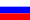# Vestnik KRAUNC. Fiz.-Mat. Nauki. 2022. vol. 40. no. 3. pp. 16–27. ISSN 2079-6641

Contents of this issue

Read Russian VersionMSC 35R11, 35A08

Research Article

On representation of solution of the diffusion equation with Dzhrbashyan-Nersesyan operators

F. T. Bogatyreva

Institute of Applied Mathematics and Automation KBSC RAS, 89 A, Shortanova str., 360000, Nalchik, Russia

E-mail: fatima_bogatyreva@bk.ru

The paper investigates a parabolic partial differential equation with fractional differentiation with respect to one of two independent variables associated with time. Such equations are usually referred to the class of fractional diffusion equations. The fractional differentiation operator is a linear combination of two Dzhrbashyan-Nersesyan operators. The main result of the work is a theorem on the general representation of regular solutions of the equation under study in an infinite strip. A fundamental solution is constructed in terms of the Wright function and its main properties are studied. In particular, formulas for fractional differentiation are proved, the asymptotic behavior is investigated, and estimates are obtained for the fundamental solution and its derivatives for large and small values of the self-similar variable, and its positiveness is proved. To construct a general solution, the Green’s function method adapted to equations containing Dzhrbashyan-Nersesyan operators is used. Particular cases of the equation under consideration include equations with Riemann-Liouville and Gerasimov-Caputo derivatives. Therefore, the results obtained remain valid for equations with these fractional differentiation operators and their combinations.

Key words: fractional diffusion equation, Dzhrbashyan-Nersesyan operators, fractional derivative, Wright function.

DOI: 10.26117/2079-6641-2022-40-3-16-27

Original article submitted: 16.10.2022

Revision submitted: 25.11.2022

For citation. Bogatyreva F. T. On representation of solution of the diffusion equation with Dzhrbashyan-Nersesyan operators. Vestnik KRAUNC. Fiz.-mat. nauki. 2022, 40: 3, 16-27.

DOI: 10.26117/2079-6641-2022-40-3-16-27

Competing interests. The authors declare that there are no conflicts of interest regarding
authorship and publication.

solely responsible for providing the final version of the article in print. The final version of the
manuscript was approved by all authors.

References

1. Dzrbashyan M. M., Nersesyan A.B. Drobnye proizvodnye i zadacha Koshi dlja differencial’nyh uravnenij drobnogo porjadka [Fractional derivatives and the Cauchy problem for fractional differential equations]. Izv. AN ArmSSR. Matem. [Izv. Acad. Sci. Arm. SSR Mat.], 1968, 3, 3–28, (In Russian).
2. Nakhushev A. M. Drobnoe ischislenie i ego primenenie [Fractional Calculus and Its Application]. Moscow, Fizmatlit, 2003, (In Russian).
3. Pskhu A. V. Solution of the first boundary value problem for a fractionalorder diffusion equation, Differential Equations, 2003, 39:9, 1359–1363, DOI:10.1023/B:DIEQ.0000012703.45373.aa
4. Pskhu A. V. Solution of boundary value problems for the fractional diffusion equation by the Green function method, Differential Equations, 2003, 39:10, 1509–1513, DOI:10.1023/B:DIEQ.0000017925.68789.e9
5. Eidelman S. D., Kochubei A. N. Cauchy problem for fractional diffusion equations, Journal of Differential Equations, 2004, 199:2, 211–255.
6. Pskhu A. V. Uravnenija v chastnyh proizvodnyh drobnogo porjadka [Fractional partial differential equations]. Moscow, Nauka, 2005, (In Russian).
7. Pskhu A. V. Uravnenie diffuzii drobnogo porjadka so mnogimi vremennymi peremennymi [Fractional diffusion equation with many time variables], Proceedings of the Third All-Russian Scientific Conference (29–31 May 2006). Part 3, Matem. Mod. Kraev. Zadachi, Samara State Technical Univ., Samara, 2006, pp. 187–190, (In Russian).
8. Luchko Yu. Boundary value problems for the generalized timefractional diffusion equation of distributed order, Fract. Calc. Appl. Anal., 2009, 12:4, 409–422.
9. Luchko Yu. Initial-boundary-value problems for the generalized multiterm time-fractional diffusion equation, J. Math. Anal. Appl., 2011, 374:2, 538–548.
10. Mamchuev M. O. Krayevyye zadachi dlya uravneniy i sistem uravneniy s chastnymi differentsialami drobnogo poryadka [Boundary value problems for equations and systems of equations with partial differentials of fractional order], Nalchik, 2013, 200 p.,(In Russian).
11. Pskhu A. V. Green functions of the first boundary-value problem for a fractional diffusionwave equation in multidimensional domains, Mathematics, 2020, 8(4), pp. 464.
12. Pskhu A. V. Stabilization of solutions to the Cauchy problem for fractional diffusion-wave equation, Journal of Mathematical Sciences, 2020, 250, 800–810.
13. Pskhu A. V., Rekhviashvili S. Fractional diffusion-wave equation with application in electrodynamics, Journal of Mathematical Sciences, 2020, 8.
14. Pskhu A. V. Boundary value problem for fractional diffusion equation in a curvilinear angle domain, Bulletin of the Karaganda Univer. Mathematics series, 2022, 1(105)/2022, 83–95.
15. Pskhu A. V. The fundamental solution of a diffusion-wave equation of fractional order, Izv. Math. 2009, 73:2, 351–392.
16. Pskhu A.V. Fractional diffusion equation with discretely distributed differentiation operator, Sib. Elektron. Mat. Izv., 2016, 13, 1078–1098.
17. Bogatyreva F. T. Boundary value problems for a first order partial differential equation with the Dzhrbashyan – Nersesyan operators, Chelyab. Fiz.-Mat. Zh., 2021, 6:4, 403–416, (In Russian).

Bogatyreva Fatima Takhirovna – Junior Researcher, Department of Fractional Calculus, Institute of Applied Mathematics and Automation, KBSC RAS, Nalchik, Russia, ORCID 0000-0003-1765-066X.